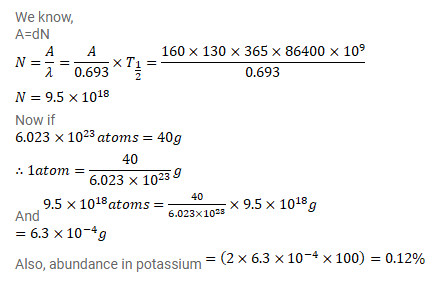# The half-life of 40K is 1.3×109y.

Question:

The half-life of ${ }^{40} \mathrm{~K}$ is $1.3 \times 10^{9} \mathrm{y}$. A sample of $1.00 \mathrm{~g}$ of pure $\mathrm{KCL}$ fives $160 \mathrm{counts} / \mathrm{s}$. Calculate the relative abundance of $40 \mathrm{~K}$ (fraction of ${ }^{40} \mathrm{~K}$ present) in natural potassium.

Solution: